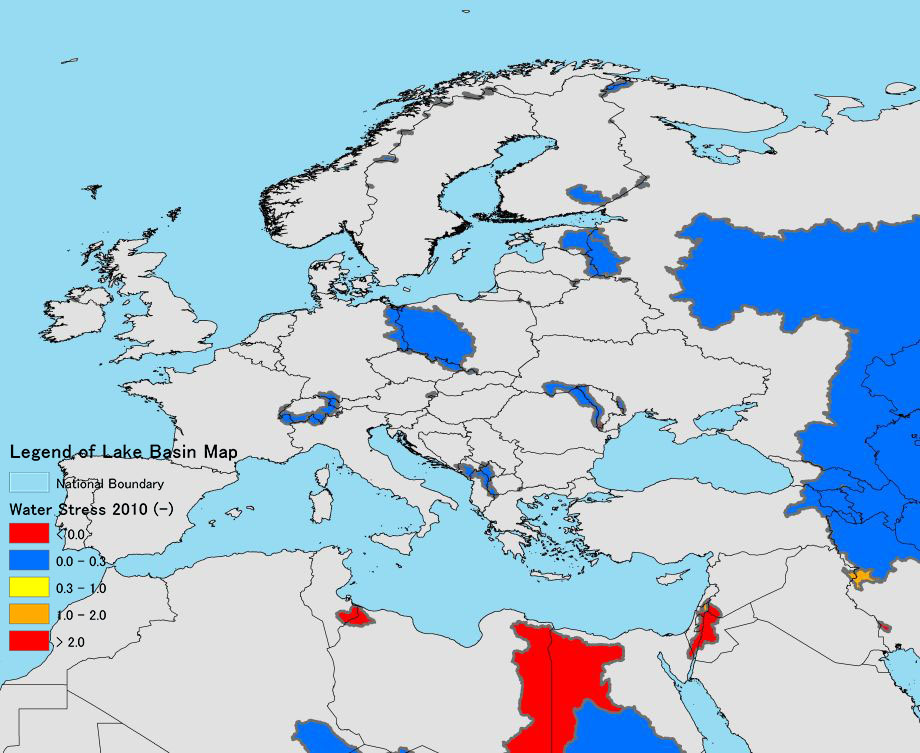The 2010 Water Stress was calculated by dividing The Water Demand by The Water Storage.

(The Water Storage was calculated assuming that the value of 1950 - 2000 was the same as the value of 2010, and by adding the monthly value to the annual value. The calculation of monthly water storage was done by subtracting actual evapotranspiration from precipitation amount. Here, the actual evapotranspiration was calculated by multiplying the possible evapotranspiration based on monthly mean temperature by the evapotranspiration ratio. The Penman formula was used for the possible evapotranspiration, and the evapotranspiration ratio was defined by the temperature designed for each climate class and by the temperature dependent regression equation.)